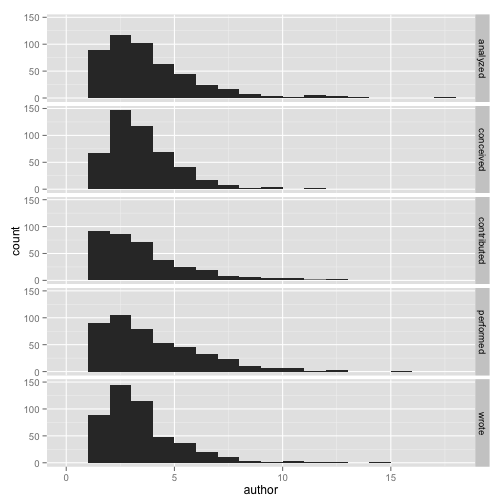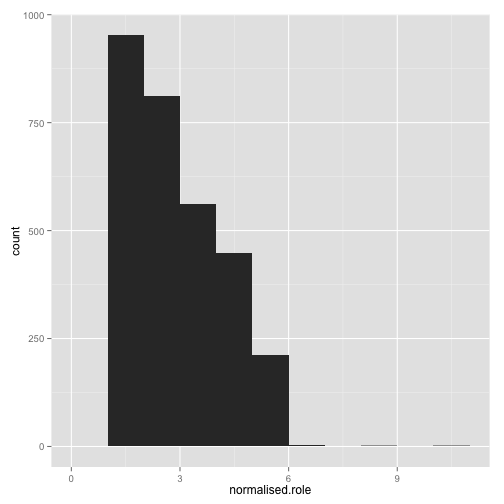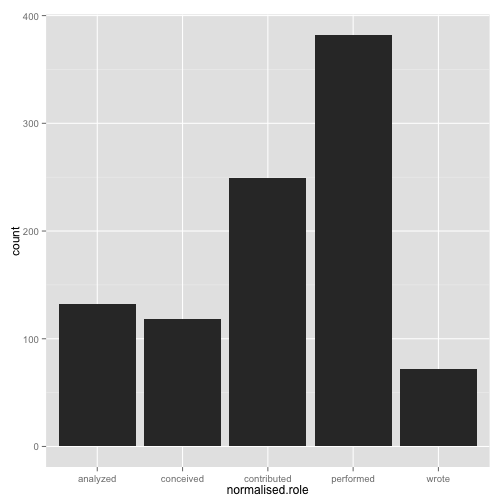# Build Relation between Author Initials and Roles

Get the data from the PLOS API:

``````# Load required packages
library(rplos)
``````
``````## Warning: package 'rplos' was built under R version 2.15.3
``````
``````## Loading required package: ggplot2
``````
``````## Warning: package 'ggplot2' was built under R version 2.15.2
``````
``````## Warning: replacing previous import 'rename' when loading 'reshape'
``````
``````## Warning: replacing previous import 'round_any' when loading 'reshape'
``````
``````##
##
## New to rplos? Tutorial at
## http://ropensci.org/tutorials/rplos_tutorial.html. Use
## suppressPackageStartupMessages() to suppress these startup messages in the
## future
``````
``````library(stringr)
library(ggplot2)

# Return DOI and author_notes for all PLOS research articles
result <- searchplos(terms = "*:*", fields = "id,author_notes", list("article_type:\"research article\"",
"doc_type:full"), limit = 1000, key = getOption("PlosApiKey"))
``````
``````## Looping - printing iterations...
``````
``````## 1
## 2
``````

Now we have to parse the author notes. The pattern we look for is role: author list. That is, we need a colon followed by a period.

``````pair.rx <- "\\s*([^:]+):\\s+([^.:]+)[.]"
pair.matches <- str_match_all(result\$author_notes, pair.rx)
author.role.table <- do.call(rbind, mapply(function(id, m) {
if (identical(m, character(0))) {
print(paste("no matches for", id))
NULL
} else {
# first column is the whole matched string
role <- m[, 2]
initials.string <- m[, 3]
stopifnot(length(role) == length(initials.string))

initials <- str_split(initials.string, "\\s+")
cbind(id = id, do.call(rbind, mapply(function(r, i) {
data.frame(role = r, author = i)
}, role, initials, SIMPLIFY = FALSE, USE.NAMES = FALSE)))
}
}, result\$id, pair.matches, SIMPLIFY = FALSE, USE.NAMES = FALSE))
``````
``````##  "no matches for 10.1371/journal.ppat.1001235"
##  "no matches for 10.1371/journal.pntd.0000349"
##  "no matches for 10.1371/journal.pntd.0000323"
##  "no matches for 10.1371/journal.pgen.0020151"
##  "no matches for 10.1371/journal.pcbi.0020051"
##  "no matches for 10.1371/journal.pcbi.0020044"
``````

# How many did we parse with this logic?

``````parsed.ids <- length(unique(author.role.table\$id))
total.ids <- nrow(result)
parsed.ids/total.ids
``````
``````##  0.988
``````

Some of the exceptions are due to different conventions. For example, the convention for PLOS Genetics seems to be

DTR, JD, TMB, MBM, and DBA conceived and designed the experiments. DTR, JD, and DBA performed the experiments. DTR, JD, LKV, and DBA analyzed the data. DTR, JD, HKT, JRF, MAP, and DBA contributed reagents/materials/analysis tools. DTR, JD, LKV, HKT, TMB, RPK, RF, MAP, NL, MBM, and DBA wrote the paper.

(from 10.1371/journal.pgen.002013)

# What if we just look at the main 5 roles?

``````main.roles <- c("Conceived and designed the experiments", "Performed the experiments",
"Analyzed the data", "Contributed reagents/materials/analysis tools", "Wrote the paper")
normalised.main.roles <- tolower(sub("^(\\w+).+\$", "\\1", main.roles))

ar.main <- transform(subset(author.role.table, tolower(gsub("\\s", "", role)) %in%
tolower(gsub("\\s", "", main.roles))), normalised.role = tolower(sub("^(\\w+).+\$",
"\\1", role)))

nrow(ar.main)/nrow(author.role.table)
``````
``````##  0.9353
``````
``````ar.main.num.authors <- aggregate(author ~ id, ar.main, length)
``````
``````ar.main.author.counts <- aggregate(author ~ id + normalised.role, ar.main, length)

m <- ggplot(ar.main.author.counts, aes(x = author))
m <- m + geom_histogram(binwidth = 1)
m + facet_grid(normalised.role ~ .)
````````````ar.main.role.counts <- aggregate(normalised.role ~ id + author, ar.main, length)

m <- ggplot(ar.main.role.counts, aes(x = normalised.role))
m + geom_histogram(binwidth = 1)
````````````authors.in.only.one.role <- subset(ar.main.role.counts, normalised.role == 1)
authors.in.only.one.role <- merge(authors.in.only.one.role[, c("id", "author")],
ar.main)

m <- ggplot(authors.in.only.one.role, aes(x = normalised.role))
m + geom_bar()
``````We can then build a smaller data frame with one row per author-paper pair and dummy variables for each of the main roles.

``````# by(ar.main, ar.main\$id, function(r) { authors <- sort(unique(r\$author))
# as.list(subset(r, select=c(author,role))) #pa.row <-
# data.frame(id=r\$id, author=authors) #for (main.role in main.roles) {
# #} });

# doi,author,conceived,performed,analyzed,contributed,wrote

# by(result, 1:nrow(result), function(r) { author.notes <- sub('[.]\$', '',
# r\$author_notes) chunks <- unlist(str_split(author.notes, '[.]\\s?'))
# if (all(str_count(chunks, ':') == 1)) { good.chunks <- str_split(chunks,
# ':\\s?') roles <- chunks[seq(1,length(chunks),by=2)] all.initials <-
# chunks[seq(2,length(chunks),by=2)] initials <- str_split(all.initials,
# '\\s+') if (length(initials) != length(roles)) { print(chunks)
# print(roles) print(initials) } names(initials) <- roles initials } else
# { NULL } #valid <- roles %in% valid.roles })

``````